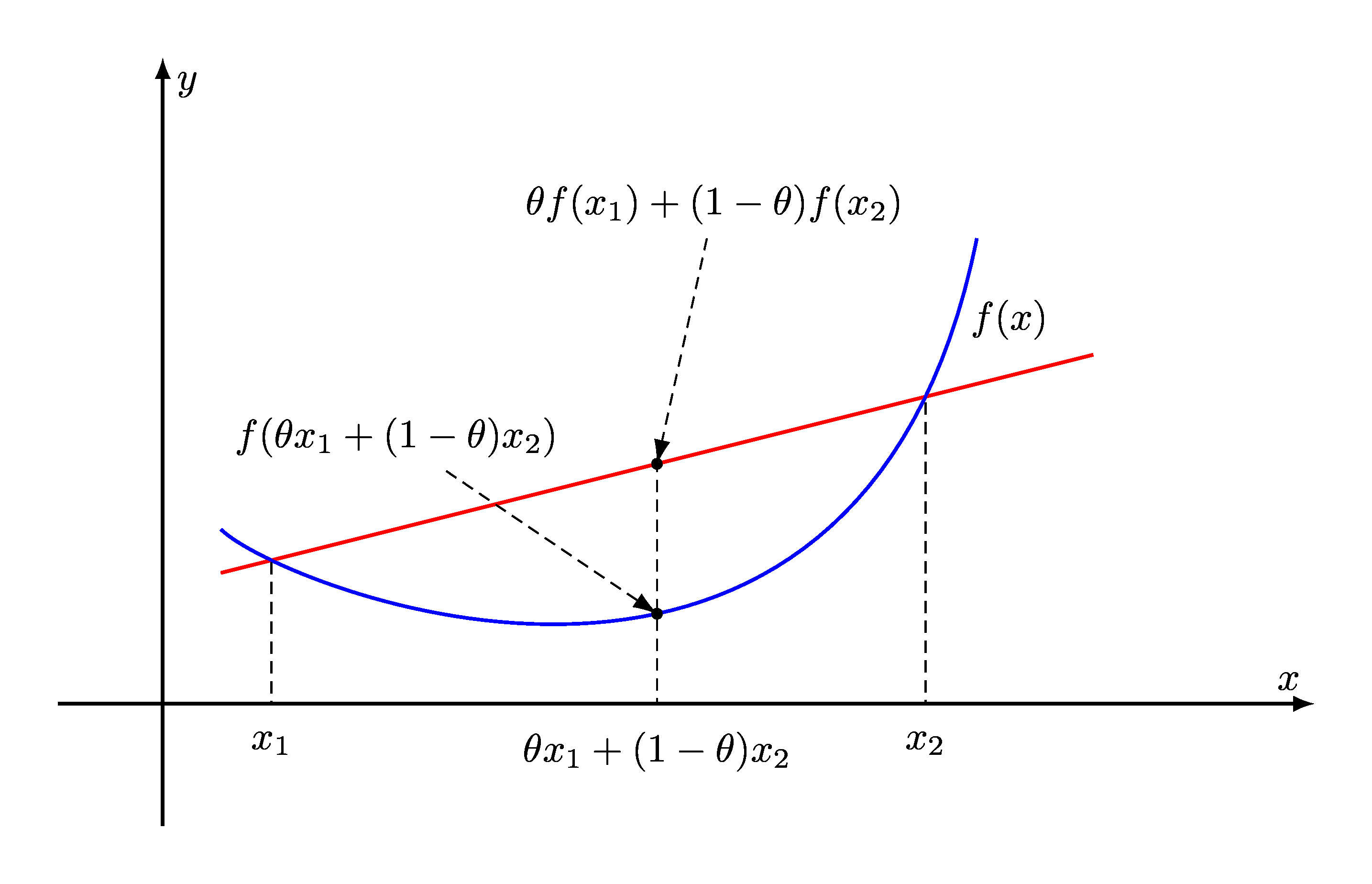# Welcome¶

## Mathematical Optimization Problems¶

A mathematical optimization problem consists of maximizing or minimizing a real valued function under a set of constraints. We shall assume $$\VV$$ to denote a finite dimensional real vector space. Typical examples of $$\VV$$ are $$\RR^n$$ and $$\SS^n$$.

Formally, we express a mathematical optimization problem as:

\begin{split} \begin{aligned} & \text{minimize} & & f_0(\bx) & & \\ & \text{subject to} & & f_i(\bx) \leq b_i, & & i = 1, \dots, m. \end{aligned} \end{split}
• $$\bx \in \VV$$ is the optimization variable of the problem.

• $$f_0 : \VV \to \RR$$ is the objective function.

• The functions $$f_i : \VV \to \RR, \; i=1,\dots, m$$ are the (inequality) constraint functions.

• The (real scalar) constants $$b_1, \dots, b_m$$ are the limits for the inequality constraints.

• A vector $$\bx \in \VV$$ is called feasible if it belongs to the domains of $$f_0, f_1, \dots, f_m$$ and satisfies all the constraints.

• A vector $$\bx^*$$ is called optimal if is feasible and has the smallest objective value; i.e. for any feasible $$\bz$$, we have $$f_0(\bz)\geq f_0(\bx^*)$$.

• An optimal vector is also called a solution to the optimization problem.

• An optimization problem is called infeasible if there is no feasible vector. i.e. there is no vector $$\bx \in \VV$$ which satisfies the inequality constraints.

• An infeasible problem doesn’t have a solution.

• A feasible problem may not have a solution if the objective function is unbounded below. i.e. for every feasible $$\bx$$, there exists another feasible $$\bz$$ such that $$f_0(\bz) < f_0(\bx)$$.

• If a feasible problem is not unbounded below, then it may have one or more solutions.

Convex Optimization focuses on a special class of mathematical optimization problems where:

• The real valued function being optimized (maximized or minimized) is convex.

• The feasible set of values for the function is a closed convex set.Fig. 1 For a convex function, the line segment between any two points on the graph of the function does not lie below the graph between the two points. Its epigraph is a convex set. A local minimum of a convex function is also a global minimum.¶

## Convex Optimization Problems¶

Convex optimization problems are usually further classified into

• Least squares

• Linear programming

• Quadratic minimization with linear constraints

• Conic optimization

• Geometric programming

• Second order cone programming

• Semidefinite programming

There are specialized algorithms available for each of these classes.

## Applications¶

Some of the applications of convex optimization include:

• Portfolio optimization

• Worst case risk analysis

• Compressive sensing

• Statistical regression

• Model fitting

• Combinatorial Optimization